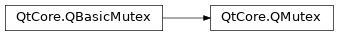# QMutex¶

The `QMutex` class provides access serialization between threads. More## Detailed Description¶

The purpose of a `QMutex` is to protect an object, data structure or section of code so that only one thread can access it at a time (this is similar to the Java `synchronized` keyword). It is usually best to use a mutex with a `QMutexLocker` since this makes it easy to ensure that locking and unlocking are performed consistently.

For example, say there is a method that prints a message to the user on two lines:

```number = 6

def method1():
number *= 5
number /= 4

def method2():
number *= 3
number /= 2
```

If these two methods are called in succession, the following happens:

```# method1()
number *= 5        # number is now 30
number /= 4        # number is now 7

# method2()
number *= 3        # number is now 21
number /= 2        # number is now 10
```

If these two methods are called simultaneously from two threads then the following sequence could result:

```# Thread 1 calls method1()
number *= 5        # number is now 30

#
# Most likely Thread 1 has been put to sleep by the operating
# system to allow Thread 2 to run.
number *= 3        # number is now 90
number /= 2        # number is now 45

number /= 4        # number is now 11, instead of 10
```

If we add a mutex, we should get the result we want:

```mutex = QMutex()
number = 6

def method1():
mutex.lock()
number *= 5
number /= 4
mutex.unlock()

def method2():
mutex.lock()
number *= 3
number /= 2
mutex.unlock()
```

Then only one thread can modify `number` at any given time and the result is correct. This is a trivial example, of course, but applies to any other case where things need to happen in a particular sequence.

When you call `lock()` in a thread, other threads that try to call `lock()` in the same place will block until the thread that got the lock calls `unlock()` . A non-blocking alternative to `lock()` is `tryLock()` .

`QMutex` is optimized to be fast in the non-contended case. A non-recursive `QMutex` will not allocate memory if there is no contention on that mutex. It is constructed and destroyed with almost no overhead, which means it is fine to have many mutexes as part of other classes.

class PySide2.QtCore.QMutex

PySide2.QtCore.QMutex(mode)

param mode:

`RecursionMode`

Constructs a new mutex. The mutex is created in an unlocked state.

Constructs a new mutex. The mutex is created in an unlocked state.

If `mode` is `Recursive` , a thread can lock the same mutex multiple times and the mutex won’t be unlocked until a corresponding number of `unlock()` calls have been made. Otherwise a thread may only lock a mutex once. The default is `NonRecursive` .

Recursive mutexes are slower and take more memory than non-recursive ones.

`lock()` `unlock()`

PySide2.QtCore.QMutex.RecursionMode

Constant

Description

QMutex.Recursive

In this mode, a thread can lock the same mutex multiple times and the mutex won’t be unlocked until a corresponding number of `unlock()` calls have been made. You should use `QRecursiveMutex` for this use-case.

QMutex.NonRecursive

In this mode, a thread may only lock a mutex once.

`QMutex()` `QRecursiveMutex`

PySide2.QtCore.QMutex.tryLock([timeout=0])
Parameters:

timeout – int

Return type:

bool

Attempts to lock the mutex. This function returns `true` if the lock was obtained; otherwise it returns `false` . If another thread has locked the mutex, this function will wait for at most `timeout` milliseconds for the mutex to become available.

Note: Passing a negative number as the `timeout` is equivalent to calling `lock()` , i.e. this function will wait forever until mutex can be locked if `timeout` is negative.

If the lock was obtained, the mutex must be unlocked with `unlock()` before another thread can successfully lock it.

Calling this function multiple times on the same mutex from the same thread is allowed if this mutex is a `recursive mutex` . If this mutex is a `non-recursive mutex` , this function will always return false when attempting to lock the mutex recursively.

`lock()` `unlock()`